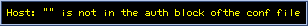# What's New?

### Click on the individual pictures or titles below to see complete pictures

NEWM51

31cm F5+ TeleConvertor(x1.7) + AO-7 + ST-8XME
L:240mim (without IR blocker)
R:60min, B:60min, (2x2 binning), SBIG filters
LRLB composit.NGC40

31cm F5+ TeleConvertor(x1.7) + AO-7 + ST-7XE
L:60mim (with IR blocker + Lumicon DSP)
R:5min, G,B:15min, (2x2 binning), SBIG filters
LRGB composit.NGC2403 (by ST-8XME)

31cm F5+ TeleConvertor(x1.7) + AO-7 + ST-8XME
L=180mim (without IR blocker), AO:25Hz
R=120min, B=120min, (2x2 binning), SBIG filters
LRLB composit.Mars 4 November 2005

14:24~14:42(GMT), 31cm F5 + ST-402XME, NP-12
R: Kenko R64, 0.1 sec x 200
G: IDAS Type-3G, 0.2 sec x 200
B: IDAS Type-3B + Fuji SP-4, 0.4 x 200
The 200 images were selected from 250 images and stacked by StellaImage5.Mars, 3 September 2005

17:34-17:49 (GMT), 31cm F5 + ST-402XME, NP-12
R: Kenko R64, 0.1 sec x 180
G: IDAS Type-3G, 0.2 sec x 180
B: IDAS Type-3B + Fuji SP-4, 0.4 x 203
The images were ranked, selected and stacked by StellaImage5.NGC4565

31cm F5+ TeleConvertor(x1.7) + AO-7 + ST-7ME
L=180mim (without IR blocker), AO:10Hz
R=30min, B=40min, (2x2 binning), SBIG filtersNGC2261: Variation of Hubble's variable nebula

31cm F5+ TeleConvertor(x1.7) + AO-7 + ST-7ME
L=60mim (with IR blocker),
RGB (2x2 binning): R=10min, G=20min, B=30min, SBIG filtersM63

31cm F5+ TeleConvertor(x1.7) + AO-7 + ST-7ME
L=120mim (w/o IR blocker), AO: 13 Hz
R=20min, B=30min, (2x2 binning), SBIG filtersM66 (NGC3627)

31cm F5+ TeleConvertor(x1.7) + AO-7 + ST-7ME
L=90mim (w/o IR blocker), AO: 8.5 Hz
RGB: by HPC-1 R=G=B=20min, SBIG RGB filtersNGC2392 (Eskimo Nebula)

31cm F5+ TeleConvertor(x1.7) + AO-7 + ST-7ME
R=60mim, G=30min, B=30min, SBIG RGB filters, AO: 8.3HzNGC2371-2

31cm F5+ TeleConvertor(x1.7) + AO-7 + ST-7ME
L (w/ IR blocker+Lumicon DeepSky filter):120min, AO: 13.7Hz
R=20mim, G=20min, B=20min, HPC-1+ SBIG RGB filtersNGC2403

31cm F5+ TeleConvertor(x1.7) + AO-7 + ST-7ME
L (w/o IR blocker):50min,
R=20mim, G=20min, B=30min (RGB: 2x2 Bin.), AO:25Hz, SBIG RGB filtersNGC7635

31cm F5+ TeleConvertor(x1.7) + AO-7 + ST-7ME
L (with SC64 + IR blocker):120min,
R=10mim, G=15min, B=20min (RGB: 2x2 Bin.), AO:18Hz, SBIG RGB filtersK648 Planetary nebula in M15

This famous PN was found in M15 globular cluster by F. G. Pease in 1928.
The size of K648 is only 2"x3" .
31cmF5 + TeleConvertor(x1.7) + AO-7 + ST-7ME
R:30min, G:10min, B:20minNGC4676 (The Mice)

31cmF5 + TeleConvertor(x1.7) + AO-7 + ST-7ME
L:(w/o filter):110min (10minx11)
False colorNGC4449

31cmF5 + TeleConvertor(x1.7) + AO-7 + ST-7ME
L:(w/o filter):100min (10minx10)
RGB; R:20min, G:20min, B:30min(20min+10min)NGC2841

31cmF5 + TeleConvertor(x1.7) + AO-7 + ST-7ME
L:(w/o filter):140min
RGB; R:20min, G:20min, B:40minNGC891

31cmF5 + TeleConvertor(x1.7) + AO-7 + ST-7ME
L:(w/o filter):110min, AO:10Hz
RGB; R:50min, G:60min, B:60min by HPC-1Go To the gallery of Images of the MARS in 2003M27 by ST-7ME

31cmF5 + TeleConvertor(x1.7) + AO-7 + ST-7E
L:(Edmound IR Blocker):40min, AO:28Hz
RGB; R:20min, G:30min, B:10min by HPC-1Seyfert's Sextet (NGC6027)
31cmF5 + TeleConvertor(x1.7) + AO-7 + ST-7E.
L(taken without IR blocker):120 min, AO: 5Hz
Clipped 35% area (4.4' x 4.4') of the original field (6.1' x 9.2').
RGB; at F=5, by ST-7E guided with ST-5;
R: 40min, G:40min, B:80min (SBIG's filter, without Binning)Jupiter; 16 Apr. 2003
19:34 (JST) , 31cm Newtonian + ST-5C with SBIG's Planet Master.
L: 5 x 0.1 sec (Kenko C4 filter + Edmound IR-blocker) ,
R: 0.1sec, G: 0.15sec, B: 0.2sec (SBIG RGB filters)

A new image processing method "Star Enhancement Processing" was developed by the collaboration between myself and AstroArts corp. Now It has been included in STELLA IMAGE 4 software (Japanese version). The star images are sharpen and enhanced. I put some examples.Core of M64

31cmF5 + TeleConvertor(x1.7) + AO-7 + ST-7E.
L(taken by R + IR bands):60 min, AO:18Hz
R: 20min, G:20min, B:40min (2x2 bin)Star burst in NGC2903

31cmF5 + TeleConvertor(x1.7) + AO-7 + ST-7E.
L(taken by R + IR bands in order to see the star burst area):120 min, AO:13Hz
R: 20min, G:20min, B:40min (2x2 bin)
Close up of the coreNGC2685 by 31cm + AO-7 + ST-7E

31cmF5 + TeleConvertor(x1.7) + AO-7 + ST-7E.
L( without IR brocker):120 min, AO:19HzHeart of M42 by 31cm + AO-7

31cmF5 + TeleConvertor(x1.7) + AO-7 + ST-7E.
L(Fuji SC-70 IR pass>700nm):180sec, AO:27Hz
RGB: IR blocker + R(Fuji SC-60):30sec,G(Fuji SP-7):60sec, B(Fuji SP-9):120sec,.AO:27Hz
The filters cover the main CCD only, in order to maximize the intensity of guide star. See the technique for LYR composit.M1(Crab nebula) by 31cm + AO-7

L: 31cm F5+ TeleConvertor(x1.7) + AO-7 + ST-7E with Edmound IR Blocker, 120min, AO:19Hz
RGB: 31cm + Teleconvertor(x1.4)+HPC-1, R=40min,G=60mim, B=80min, SBIG RGB filter
Processed by DDP and USM. The L-R deconvolution has not been used. (Revised on 15 Oct. 2002)

L: 31cm F5+ TeleConvertor(x1.7) + AO-7 + ST-7E with Edmound IR Blocker, 90min, AO:27Hz
RGB: 31cm + Teleconvertor(x1.4)+HPC-1, R=G=40mim, B=80min, SBIG RGB filter
One iteration by CCDSharp L-R deconvolution "after" DDP.

L: 31cm F5+ TeleConvertor(x1.7) + AO-7 + ST-7E with Edmound IR Blocker, 60min, AO:5Hz
RGB: 31cm + Teleconvertor(x1.4)+HPC-1, R=20mim, G=30min, B=10min, SBIG RGB filter
The nebula part has been composed on the L-ch of wide view image by HPC-1.NGC7662

31cm F5 + TeleConvertor(x1.7) + AO-7 + ST-7E
L=10min, R=G=B=5min(Tokai Type III).

Left: C8 +AO-7+ST-7E , reprocessed with CCDSharp
L: 20min (5min x 4) , R=G=5min and B=10min,with Tokai LRGB Type-3 filter.
Right: 31cm F5 + TeleConvertor (F=8.5) + AO-7 + ST-7E
L:60min(5min x 12), R=G=5min, B=10min.NGC6826 by 31cm Newtonian

31cm F5 + x1.7 tele-convertor + AO-7 + ST-7E
L=27.5min, R=G=B=10minM57 by 31cm Newtonian

31cm F5 + x1.7 tele-convertor + AO-7 (10Hz)+ ST-7E
L=35min, R=10min, G=15min, B=20minM97 by 31cm Newtonian

L=90min (with x1.7 tele-convertor + AO-7 + ST-7E)
R=B=G=60min (with x1.4 tele-convertor) + HPC-1M82 by 31cm Newtonian + a new tele-convertor for AO-7
L: 40 min (witn x 1.7 tele-convertor + AO-7 + ST-7E)
R=40min, G=60 min, B=60 min (with x1.4 tele- convertor) + HPC-1
where a new tele-convertor for AO-7 was used.Horse Head Nebula re-processed
L: 180min (20min x 9 with R64 + Edmound IR block)) , R=G=60min, B=80min,
31cm +HPC-1, guided by ST-5. Compare the images of stars with those in the previous one.

•